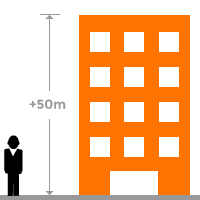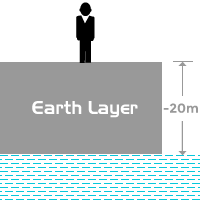IntegerA number, which represents a real whole quantity by either positive sign or negative sign is defined as an integer.

Integers are also whole numbers and represents whole quantities but it represents whole quantities with both positive and negative signs. The positive or negative sign before the whole number indicates the direction. If the direction is natural, the whole number is appeared with positive sign. If the direction is opposite to actual direction, the whole number is appeared with negative sign. The whole number zero does not contain either of them.

However, all the integers are not whole numbers and natural numbers but all natural and whole numbers are integers.

How to use

Consider a building and assume the height of the building is $50$ meters. Actually, the height of the building is measured from the surface of the earth to top of the building. This direction is considered as positive. So, $+50$ $m$ or $50$ $m$ represents the height of the building.Consider another case. Assume, the ground water is $20$ meters deep from the surface of the earth. We cannot measure the depth from surface of the ground water to surface of the earth. It should be measured from surface of the water to surface of the water in downwards. The direction of measuring in this case is opposite to the direction of the actual measuring. Therefore, the direction of measuring depth is considered as negative. So, $–20$ $m$ represents depth from the surface of the earth.

Representation

So, the collection whole numbers with both positive and negative numbers form a set of integers. The set of integers is denoted by either $Z$ or $I$ in number system and the set of integers are written in set form in mathematics as follows.

$Z$ (or) $I=\left\{\dots ,–3,–2,–1,0,1,2,3,\dots \right\}$

Integers start from zero and continue infinitely in positive signs and also continue with negative signs. So, the set of integers is an example for an infinite set.

Latest Math Topics
Latest Math Problems
Email subscription
Math Doubts is a best place to learn mathematics and from basics to advanced scientific level for students, teachers and researchers. Know more